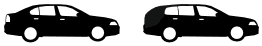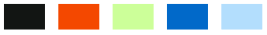Basic Principle of CountingFundamental Principle of Counting

Let us consider the following problem. Dipesh has 3 pants and 2 shirts. How many different pairs of a pant and a shirt, can he dress up with? There are 3 ways in which a pant can be chosen because there are 3 pants available. Similarly, a shirt can be chosen in 2 ways. For every choice of a pant, there are 2 choices of a shirt. Therefore, there are 3 × 2 = 6 pairs of a pant and a shirt.

Let us name the three pants as P1 , P2, P3 and the two shirts as S1 , S2 . Then, these six possibilities can be illustrated in Figure 7.1.Let us consider another problem of the same type. DIpesh has 2 school bags, 3 tiffin boxes, and 2 water bottles. In how many ways can he carry these items (choosing one each). A school bag can be chosen in 2 different ways. After a school bag is chosen, a tiffin box can be chosen in 3 different ways. Hence, there are 2 × 3 = 6 pairs of school bags and a tiffin box. For each of these pairs, a water bottle can be chosen in 2 different ways. Hence, there are 6 × 2 = 12 different ways in which, DIpesh can carry these items to school. If we name the 2 school bags as B1, B2, the three tiffin boxes as T1, T2, T3, and the two water bottles as W1, W2, these possibilities can be illustrated in Fig. 7.2.In fact, the problems of the above types are solved by applying the following a principle known as the fundamental principle of counting, or, simply, the multiplication the principle, which states that “If an event can occur in m different ways, following which another event can occur in n different ways, then the total number of occurrence of the events in the given order is m×n.”

The above principle can be generalized for any finite number of events. For example, for 3 events, the principle is as follows: ‘If an event can occur in m different ways, following which another event can occur in n different ways, following which a third event can occur in p different ways, then the total number of occurrence to ‘the events in the given order is m × n × p.” In the first problem, the required number of ways of wearing a pant and a shirt was the number of different ways of the occurrence of the following events in succession:

(i) the event of choosing a pant
(ii) the event of choosing a shirt.

In the second problem, the required number of ways was the number of different ways of the occurrence of the following events in succession:

(i) the event of choosing a school bag
(ii) the event of choosing a tiffin box
(iii) the event of choosing a water bottle.

Here, in both cases, the events in each problem could occur in various possible orders. But, we have to choose any one of the possible orders and count the number of different ways of the occurrence of the events in this chosen order.

Example:   A coin is tossed, a die is rolled, and a card is drawn from a standard deck. How many outcomes are possible?
Notice that a tree diagram for this problem would take a lot of time to draw out with all the possibilities. So, we apply the Fundamental Counting Principle to count all the ordered triplets for the coin, die, and card such as
(H, 3, ace of hearts) or (T, 2, queen of clubs).
n(coin)  n(die)  n(card) = 2  6  52 = 624
There would 624 possible outcomes when a coin is tossed, a die is rolled, and a card is drawn.

Example: You are buying a new car.

 There are 2 body styles:sedan or hatchback There are 5 colors available:There are 3 models: GL (standard model),SS (sports model with bigger engine)SL (luxury model with leather seats)

How many total choices?

You can see in this "tree" diagram:You can count the choices, or just do the simple calculation:

Total Choices = 2 × 5 × 3 = 30

• Independent or Dependent?

But it only works when all choices are independent of each other.

If one choice affects another choice (i.e. depends on another choice), then a simple multiplication is not right.

Example: You are buying a new car ... but ...

the salesman says "You can't choose black for the hatchback" ... well then things change!You now have only 27 choices.

Because your choices are not independent of each other.

But you can still make your life easier with this calculation:

Choices = 5×3 + 4×3 = 15 + 12 = 27